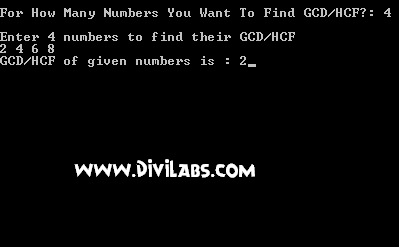# C Program Code For Finding HCF / GCD of N entered numbers (Highest Common Factor == Greatest Common Divisor)

/* The following C Program returns the HCF/GCD of N entered numbers. It uses an array to stored the numbers entered by users. The program will display GDC/HCF not exist when a character is entered instead of an integer */

#include<stdio.h>
#include<conio.h>

int n=0;
int HCF(int *x)
{
int a,c,d,m=0,i=1,tt=0,t=0;
while(tt<n)
{
a[tt]=*x;
x++;
tt++;
}
while(t<n)
{
if(a[t]==0)
{
printf("\n GCD/HCF of the given numbers is : 0");
goto a;
}
t++;
}
while(m<n)
{
while(i<n)
{
if(a[m]>a[i])
{
c=a[m];
a[m]=a[i];
a[i]=c;
}
i++;
}
m++;
}
c=a;
d=n-1;
while(c>1)
{
while(a[d]%c==0)
{
if(d>1)
{
d--;
continue;
}
else
return c;
}
c--;
if(c==1)
return -1;
}
a:
return -2;
}

int main()
{
int x,z=0;
printf("\nFor How Many Numbers You Want To Find GCD/HCF?: ");
scanf("%d",&n);
printf("\nEnter %d numbers to find their GCD/HCF\n", n);
while(z<n)
{
scanf("%d",&x[z]);
z++;
}

x[n]=HCF(x);
if(x[n]==-1)
{
printf("\n HCF/GCD doesn't exist");
}
else if(x[n]==-2)
{
printf("\nThe GCD/HCF of given numbers is : 1");
}
else
{
printf("GCD/HCF of given numbers is : %d",x[n]);
}
getch();
return 0;
}

Screenshots: